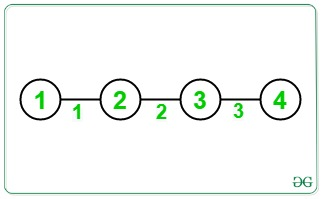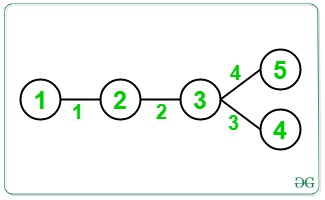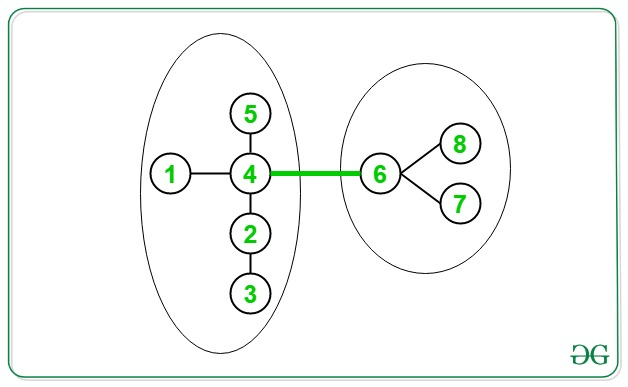Related Articles

# Count number of times each Edge appears in all possible paths of a given Tree

• Difficulty Level : Hard
• Last Updated : 07 Sep, 2021

Given an Undirected Connected Graph in the form of a tree consisting of N nodes and (N – 1) edges, the task for each edge is to count the number of times it appears across all possible paths in the Tree.

Examples:

Input:Output: 3 4 3
Explanation:
All possible paths of a given tree are {(1, 2), (1, 3), (1, 4), (2, 3), (2, 4), (3, 4)}
Edge 1 occurs in the paths {(1, 2), (1, 3), (1, 4)}. Therefore, the frequency of the edge is 3.
Edge 2 occurs in the paths {(1, 3), (1, 4), (2, 3), (2, 4)}. Therefore, the frequency of the edge is 4.
Edge 3 occurs in the paths {(1, 4), (2, 4), (3, 4)}. Therefore, the frequency of the edge is 3.

Input:Output: 4 6 4 4
Explanation:
Edge 1 occurs in the paths {(1, 2), (1, 3), (1, 4), (1, 5)}. Therefore, the frequency of the edge is 4
Edge 2 occurs in the paths {(1, 3), (1, 4), (1, 5), (2, 3), (2, 4), (2, 5)}. Therefore, the frequency of the edge is 6
Edge 3 occurs in the paths {(1, 4), (2, 4), (3, 4), (4, 5)}. Therefore, the frequency of the edge is 4
Edge 4 occurs in the paths {(1, 5), (2, 5), (3, 5), (4, 5)}. Therefore, the frequency of the edge is 4

Naive Approach: The simplest approach is to generate all possible paths from each node of the given graph and store the count of edges occurring in these paths by a HashMap. Finally, print the frequencies of each edge.

Time Complexity: O(N2
Auxiliary Space: O(N)

Efficient Approach: To optimize the above approach, the following observation needs to be made:The green-colored edge will appear in all the paths that connect any vertex from the subtree on its left to any vertex from the subtree on its right.
Therefore, the number of paths in which the edge occurs = Product of the count of nodes in the two subtrees = 5 * 3 = 15.

Follow the steps below in order to solve the problem:

• Root the tree at any random vertex, say 1.
• Perform DFS at Root. Using DFS calculate the subtree size connected to the edges.
• The frequency of each edge connected to subtree is (subtree size) * (N – subtree size).
• Store the value calculated above for each node in a HashMap. Finally, after complete the traversal of the tree, traverse the HashMap to print the result.

Below is the implementation of the above approach:

## C++

 `// C++ Program to implement``// the above approach``#include ``using` `namespace` `std;` `// Number of nodes``int` `N;` `// Structure of a Node``struct` `Node {``    ``int` `node;``    ``int` `edgeLabel;``};` `// Adjacency List to``// represent the Tree``vector adj;` `// Stores the frequencies``// of every edge``vector<``int``> freq;` `// Function to perform DFS``int` `dfs(``int` `u = 1, ``int` `p = 1)``{``    ``// Add the current node to``    ``// size of subtree rooted at u``    ``int` `sz = 1;` `    ``// Iterate over its children``    ``for` `(``auto` `a : adj[u]) {` `        ``// Check if child is not parent``        ``if` `(a.node != p) {` `            ``// Get the subtree size``            ``// for the child``            ``int` `val = dfs(a.node, u);` `            ``// Set the frequency``            ``// of the current edge``            ``freq[a.edgeLabel]``                ``= val * (N - val);` `            ``// Add the subtree size``            ``// to itself``            ``sz += val;``        ``}``    ``}` `    ``// Return the subtree size``    ``return` `sz;``}` `// Function to add edge between nodes``void` `addEdge(``int` `u, ``int` `v, ``int` `label)``{``    ``adj[u].push_back({ v, label });``    ``adj[v].push_back({ u, label });``}` `// Function to print the frequencies``// of each edge in all possible paths``void` `printFrequencies()``{` `    ``// Stores the frequency``    ``// of all the edges``    ``freq = vector<``int``>(N);` `    ``// Perform DFS``    ``dfs();` `    ``for` `(``int` `i = 1; i < N; i++) {``        ``cout << freq[i] << ``" "``;``    ``}``}` `// Driver Code``int` `main()``{``    ``N = 4;``    ``addEdge(1, 2, 1);``    ``addEdge(2, 3, 2);``    ``addEdge(3, 4, 3);` `    ``printFrequencies();` `    ``return` `0;``}`

## Java

 `// Java Program to implement``// the above approach``import` `java.util.*;``class` `GFG{` `// Number of nodes``static` `int` `N;` `// Structure of a Node``static` `class` `Node``{``    ``int` `node;``    ``int` `edgeLabel;``    ``public` `Node(``int` `node, ``int` `edgeLabel)``    ``{``        ``super``();``        ``this``.node = node;``        ``this``.edgeLabel = edgeLabel;``    ``}``};` `// Adjacency List to``// represent the Tree``static` `Vector []adj = ``new` `Vector[``100005``];` `// Stores the frequencies``// of every edge``static` `int` `[]freq;` `// Function to perform DFS``static` `int` `dfs(``int` `u , ``int` `p)``{ ``    ``// Add the current node to``    ``// size of subtree rooted at u``    ``int` `sz = ``1``;` `    ``// Iterate over its children``    ``for` `(Node a : adj[u])``    ``{``        ``// Check if child is not parent``        ``if` `(a.node != p)``        ``{``            ``// Get the subtree size``            ``// for the child``            ``int` `val = dfs(a.node, u);` `            ``// Set the frequency``            ``// of the current edge``            ``freq[a.edgeLabel] = val * (N - val);` `            ``// Add the subtree size``            ``// to itself``            ``sz += val;``        ``}``    ``}` `    ``// Return the subtree size``    ``return` `sz;``}` `// Function to add edge between nodes``static` `void` `addEdge(``int` `u, ``int` `v, ``int` `label)``{``    ``adj[u].add(``new` `Node( v, label ));``    ``adj[v].add(``new` `Node( u, label));``}` `// Function to print the frequencies``// of each edge in all possible paths``static` `void` `printFrequencies()``{` `    ``// Stores the frequency``    ``// of all the edges``    ``freq = ``new` `int``[N];` `    ``// Perform DFS``    ``dfs(``1``, ``1``);` `    ``for` `(``int` `i = ``1``; i < N; i++)``    ``{``        ``System.out.print(freq[i] + ``" "``);``    ``}``}` `// Driver Code``public` `static` `void` `main(String[] args)``{``    ``N = ``4``;``    ``for` `(``int` `i = ``0``; i < adj.length; i++)``        ``adj[i] = ``new` `Vector();``    ``addEdge(``1``, ``2``, ``1``);``    ``addEdge(``2``, ``3``, ``2``);``    ``addEdge(``3``, ``4``, ``3``);``  ` `    ``printFrequencies();``}``}` `// This code is contributed by shikhasingrajput`

## Python3

 `# Python3 program to implement``# the above approach` `# Number of nodes``N ``=` `4` `# Structure of a Node``class` `Node:``    ` `    ``def` `__init__(``self``, v, label):``        ` `        ``self``.node ``=` `v``        ``self``.edgeLabel ``=` `label``        ` `# Adjacency list to``# represent the Tree``adj ``=` `[]``for` `i ``in` `range``(``100005``):``    ``adj.append([])` `# Stores the frequencies``# of each edge``freq ``=` `[``0``] ``*` `N` `# Function to perform DFS``def` `dfs(u ``=` `1``, p ``=` `1``):``    ` `    ``global` `N``    ` `    ``# Add the current node to``    ``# size of subtree rooted at u``    ``sz ``=` `1``    ` `    ``# Iterate over its children``    ``for` `a ``in` `adj[u]:``        ` `        ``# Check if child is not parent``        ``if` `a.node !``=` `p:``            ` `            ``# Get the subtree size``            ``# for the child``            ``val ``=` `dfs(a.node, u)``            ` `            ``# Set the frequency``            ``# of the current edge``            ``freq[a.edgeLabel] ``=` `val ``*` `(N ``-` `val)``            ` `            ``# Add the subtree size``            ``# to itself``            ``sz ``+``=` `val``            ` `    ``# Return the subtree size``    ``return` `sz` `# Function to add edge between nodes``def` `addEdge(u, v, label):``    ` `    ``adj[u].append(Node(v, label))``    ``adj[v].append(Node(u, label))``    ` `# Function to print the frequencies``# of each edge in all possible paths``def` `printFrequencies():``    ` `    ``# Stores the frequency``    ``# of all the edges``    ``global` `N``    ` `    ``# Perform DFS``    ``dfs()``    ` `    ``for` `i ``in` `range``(``1``, N):``        ``print``(freq[i], end ``=` `" "``)``    ` `# Driver code``N ``=` `4``addEdge(``1``, ``2``, ``1``)``addEdge(``2``, ``3``, ``2``)``addEdge(``3``, ``4``, ``3``)` `printFrequencies()``    ` `# This code is contributed by Stuti Pathak`

## C#

 `// C# Program to implement``// the above approach``using` `System;``using` `System.Collections.Generic;``class` `GFG{` `// Number of nodes``static` `int` `N;` `// Structure of a Node``public` `class` `Node``{``  ``public` `int` `node;``  ``public` `int` `edgeLabel;``  ``public` `Node(``int` `node,``              ``int` `edgeLabel)``  ``{``    ``this``.node = node;``    ``this``.edgeLabel = edgeLabel;``  ``}``};` `// Adjacency List to``// represent the Tree``static` `List []adj =``       ``new` `List;` `// Stores the frequencies``// of every edge``static` `int` `[]freq;` `// Function to perform DFS``static` `int` `dfs(``int` `u, ``int` `p)``{ ``    ``// Add the current node to``    ``// size of subtree rooted at u``    ``int` `sz = 1;` `    ``// Iterate over its children``    ``foreach` `(Node a ``in` `adj[u])``    ``{``        ``// Check if child is not parent``        ``if` `(a.node != p)``        ``{``            ``// Get the subtree size``            ``// for the child``            ``int` `val = dfs(a.node, u);` `            ``// Set the frequency``            ``// of the current edge``            ``freq[a.edgeLabel] = val * (N - val);` `            ``// Add the subtree size``            ``// to itself``            ``sz += val;``        ``}``    ``}` `    ``// Return the subtree size``    ``return` `sz;``}` `// Function to add edge between nodes``static` `void` `addEdge(``int` `u, ``int` `v,``                    ``int` `label)``{``  ``adj[u].Add(``new` `Node(v, label));``  ``adj[v].Add(``new` `Node(u, label));``}` `// Function to print the frequencies``// of each edge in all possible paths``static` `void` `printFrequencies()``{``  ``// Stores the frequency``  ``// of all the edges``  ``freq = ``new` `int``[N];` `  ``// Perform DFS``  ``dfs(1, 1);` `  ``for` `(``int` `i = 1; i < N; i++)``  ``{``    ``Console.Write(freq[i] + ``" "``);``  ``}``}` `// Driver Code``public` `static` `void` `Main(String[] args)``{``  ``N = 4;``  ``for` `(``int` `i = 0; i < adj.Length; i++)``    ``adj[i] = ``new` `List();``  ``addEdge(1, 2, 1);``  ``addEdge(2, 3, 2);``  ``addEdge(3, 4, 3);``  ``printFrequencies();``}``}` `// This code is contributed by gauravrajput1`

## Javascript

 ``
Output:
`3 4 3`

Time Complexity: O(N)
Auxiliary Space: O(N)

Attention reader! Don’t stop learning now. Get hold of all the important DSA concepts with the DSA Self Paced Course at a student-friendly price and become industry ready.  To complete your preparation from learning a language to DS Algo and many more,  please refer Complete Interview Preparation Course.

In case you wish to attend live classes with experts, please refer DSA Live Classes for Working Professionals and Competitive Programming Live for Students.

My Personal Notes arrow_drop_up